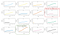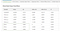# Mixed Effects Models

## Analysing chick weights over time under four different diet categories using AutoStat®

In this tutorial we will use a linear mixed effects model and compare it to a standard linear regression.

The usual representation of the linear regression is given as:

Y=Xβ+ϵ

where Y is the outcome measure, X is a design matrix of explanatory variables, β are the estimated coefficients of the model and the residual (error) term is represented as ϵ.

The assumptions of a linear regression include:

• The data are independent samples
• The errors are distributed as N(0,σ²)

We now consider a situation where data are taken longitudinally as a growth study. For such data, there are repeated measures of individuals, and often the variance around the growth profiles differs over time (not heterogeneous).

Conceptually, it is easy to understand why heterogeneity of errors occurs in growth profiles. Imagine a situation of human children being monitored, from birth, for weight over a 5 year period. When we are born, there is only a small variance in birth weights, as by necessity, we are small when we are born. From birth, each individual will follow their own individual “growth curve”, due to factors such as genetics, environment and diet. Our growth is not exactly on-target with such a profile, and we may vary either side of our individual “mean growth” at any time. Additionally, while we may start life with a variation of say 10% between weights, as we grow, a 10% difference in weights will also increase in range, therefore, it would not be possible to maintain the same range of values as we grow.

In this study, we will look at the growth of poultry chicks over time. These chicks are raised in a similar environment, but are subject to 4 different diets, with diet allocation being assigned randomly at birth.

# Data Visualisation

Here is the mean profile of Weight by diet over time. This profile looks like a potential candidate for a spline on the time variable, with growth rates increasing at around the 12 weeks of age, and possibly also at 6 weeks.

If you do a scatterplot, instead of the mean profile, you can immediately see that the variance around a fitted profile will not be constant, with variance increasing as the chicks grow.

From the plot we note that Diet 1 seems to have the lowest weights for the chicks, but the other diets are not clear as to whether they are diffferent. We also notice a particular chick in Diet 2 (purple dots) that has very low body weight compared to the other chicks in its treatment group.

In this particular set of measurements, not all chicks are measured at each time. A quick way to check this is using sub-groups in the Visualisation module. Here is an example plot, and we note feature like chick 18 dropping out of the study early, chick 15 not thriving and dropping out halfway through the study, and chick 21 growing quite quickly.Fig 3. Line plot of individual chick weights over time. Note the thriving growth from Chick #1, #2, #14 and #21 versus Chick #15, #16, #18.

Here is the same plot, but placed on the 4 separate charts for diet responses. We do this using by plotting each Chick ID as a sub-group, and each Diet category in the sub-plot:

# Linear Regression

We firstly conducted a linear regression using a Bayesian approach with variable selection using a spike-slab G-prior.

Need to brush up on linear regression with Bayesian variable selection? In our last tutorial, we explored three different Linear Regression approaches: Frequentist, Lasso and Bayesian Variable Selection. You can access this here.

The variable selection aspect of this model reflects what we would intuitively think should occur. None of the main effects for diet are shown to have an inclusion probability above 0.15. This makes sense, as the chicks at birth have not been subjected to the diet treatments, and are yet to be randomly assigned to the treatment. If the random assignment is sucessful, these “intercept” terms should not contribute to the model in any significant way.

Therefore we have the constant term, which in this case is essentially the mean birth weight, an effect of time, as the chicks grow, and an effect of each diet over the standard diet through time. This model only compares each diet to the base diet (Diet 1), not to each other.

The posterior distributions of the coefficients (below) have been produced using a model average approach. In summary, each coefficient is a product of the coefficient values it takes over each of the models, and weighted on how likely the model is. Therefore, terms that are in the most probable models are given more weight than those which occur in few or less probable models. Terms which appear in only improbable models tend to be shrunk towards zero (0).

We see that the main effects of diet are generally small in magnitude, with the exception of Diet 3, however, this term has large standard deviation, so it is not significant.

The coefficients for Time (and interactions with Diet) are more substantive, with low standard deviation. We see that the coefficient for time indicates that for each week under Diet 1, chicks would gain, on average, 7 grams in weight, and under the other diet regimes, this gain would be greater.

We also note that the coefficients are not sensible in several aspects. For example,

• The constant does not reflect the weight of chicks at birth, with it underestimating the birth weight by around 10 grams.
• The estimated sigma is very large, and not reflective of chicks when they are young.

The first issue would be improved by including a spline term for Time, but the issue with the mean-variance relationship will not be addressed by using the linear regression framework.

The Actual vs. Prediction plot shows the under-estimation of the weight at birth. You can find this in the diagnostics tab in the Results module.

We can also see the large range of the residuals in the model via the histogram in the diagnostics panel, with some residuals in the order of ±100 grams.

# Mixed Models

The linear mixed effects model is represented as:

Here, i is an individual chick, Xi is the design matrix of the fixed effects for chick i (as we are familiar with from the standard linear regression), β are the coefficients for the fixed effects and the error term ϵ_i is distributed as N(0,σ²).

The big change is the addition of a second design matrix Zi and the subject specific random effects b_i. These b_i allow us to model the subject specific means and enable us to capture the marginal dependence among the observations on the i-th unit.

The distribution of the random effects is b ∼ N(0,D). The extra variance component D is considered independent of the residual variance (σ²). The analyst has some options for modelling the elements of D. They can be independent between b’s, or there can be a variance-covariance structure between some or all of the b’s.

Lets start by doing a linear mixed model with a spline regression for Time. The model will be:

Weight =

Constant or Intercept + Diet + Time + Diet:Time + ChickID + ChickID:Time

where terms involving Chick are considered to be random.

We can see from the output, that many improvements in fit have been made with this model. For example, the Constant is now at a much more reasonable estimate, around 10 grams heigher than the linear regression. This is due to using a spline term in time, not necessarily due to mixed effects modelling. We can see the effect of the spline term by viewing the variable impact charts tab:

We see that growth is linear until around 6–8 weeks, and then the slope of the growth changes (but remains fairly linear). The variable impact charts also shed light on the effect of diet over time, with each diet type indicated as having an additional effect on growth (chickens weighing more over time), with Diet 3 having a very strong effect on final growth weight.

Looking at the standard output tab we notice that the model is probably subject to overfitting (see below). Biologically, we know that chicks are allocated randomly to the Diet groups, so the terms for Diet are probably not contributing to the model in a meaningful way.

Looking at the Diagnostics Tab we now see residuals that are much improved, with a range now reduced to ±20 grams, and the actual vs predicted graph no longer shows the increasing mean-variance relationship of the linear model.

## Model averaging and overfitting

To try and reduce the possibility of overfitting, we will now use a Normal spike slab prior on the fixed effects. AutoStat® currently provides both a Normal spike slab and a G-prior spike slab for variable selection. Generally, these models will give similar inferences, particularly when using value priors for the “slab” component of the model.

The results given in the Stochastic search panel indicate that the main effect of Diet (birth weight for each diet) is very much reduced, with inclusion probabilities of 5% or less.

The first model in the Table has a model probability of 0.83, and includes all of the spline terms, and none of the main effect Diet coefficients. The high model probability indicates that the coefficients returned for main effects diet will have shrinkage towards zero (0).

Here is the table of regression coefficients determined using model averaging, where we can see that these terms for Diet have been subjected to shrinkage, and now are of much lower magnitude than in the previous model.Fig 14. Mixed Model (Normal Spike Slab) — Note shrinkage of Diet variables combatting overfitting.

# Summary

In this tutorial we have explored using splines for non-linear effects in linear regression, and the use of mixed models for repeated measures growth profiles. We then used variable selection to shrink non-required terms in the model.

We found that:

• Spline terms on non-linear response resulted in better fits, particularly at the intercept;
• Mixed effects models resulted in improved residual variances, as they accounted for variance attributable;
• Variable selction using model averaging resulted in unimportant terms having shrinkage applied to their estimators.

# See for yourself

Want to start running your own statistics and data science projects?

Head to our website for a free trial here:

Https://autostat.com.au

Written by

## More From Medium

Welcome to a place where words matter. On Medium, smart voices and original ideas take center stage - with no ads in sight. Watch
Follow all the topics you care about, and we’ll deliver the best stories for you to your homepage and inbox. Explore
Get unlimited access to the best stories on Medium — and support writers while you’re at it. Just \$5/month. Upgrade